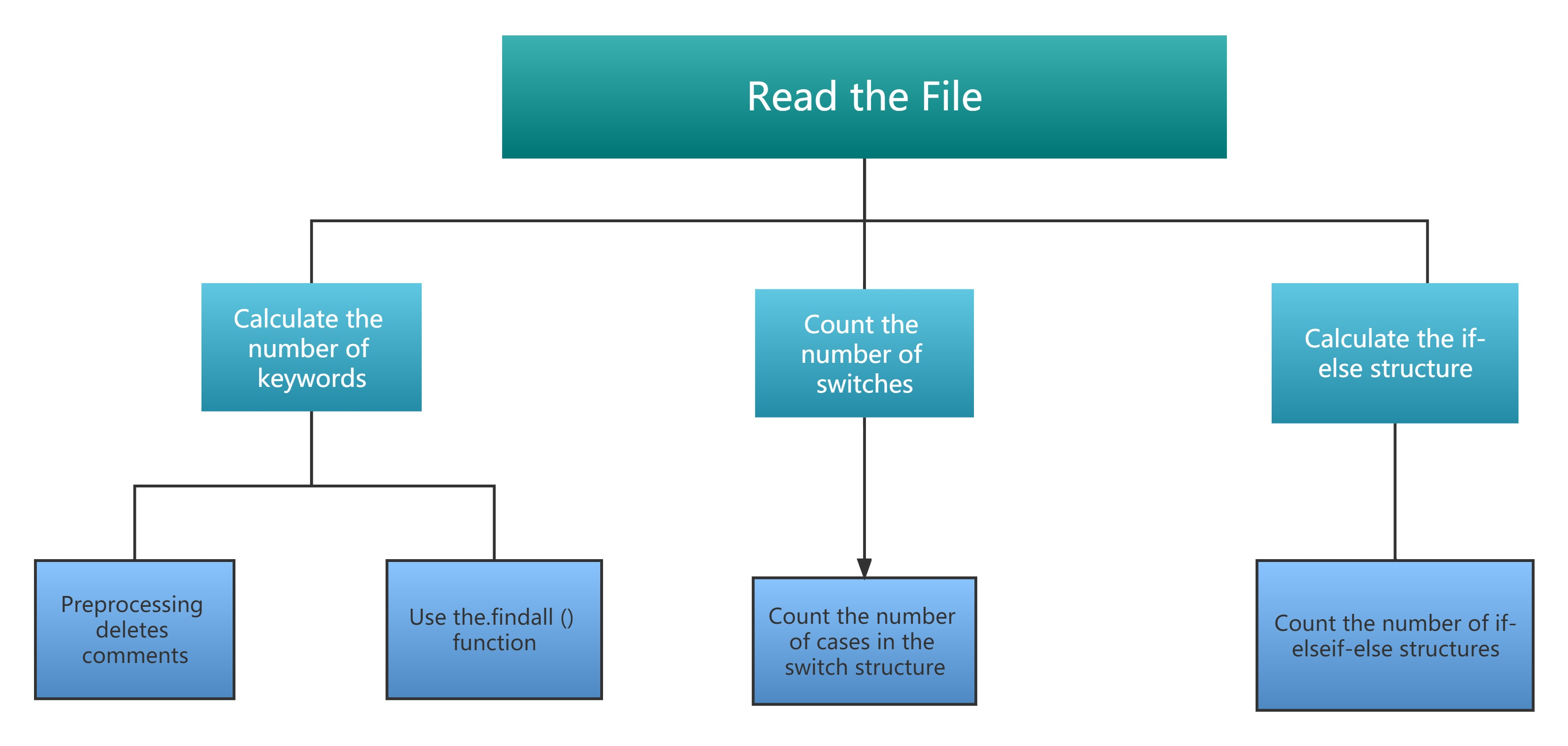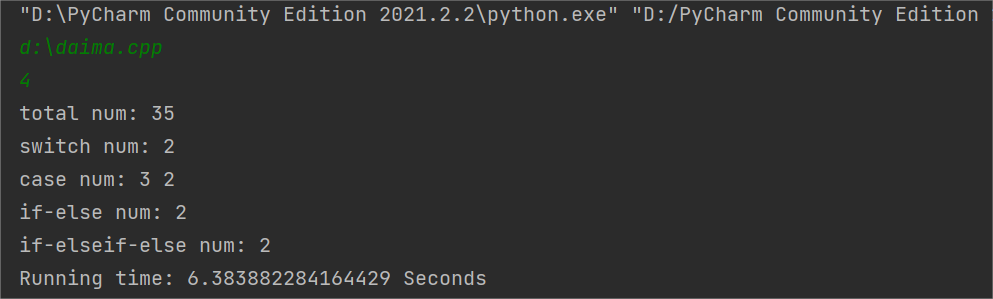# EE308 LAB1-2

The Link of Requirement of This Assignmenthttps://bbs.csdn.net/topics/608734907
The Aim of This AssignmentGitHub Using & Keywords Counting
MU STU ID and FZU STU ID20124805_832001130
githubhttps://github.com/Blue-Ice03/EE308-LAB1_2

## 1. PSP TABLE

PSP2.1
Personal Software Process Stages

Planning

· Estimate· 估计这个任务需要多少时间
10
15
Development

· Analysis· 需求分析 (包括学习新技术)
40
60
· Design Spec· 生成设计文档
10
10
· Design Review· 设计复审 (和同事审核设计文档)
20
20
· Coding Standard· 代码规范 (为目前的开发制定合适的规范)
30
30
· Design· 具体设计
80
60
· Coding· 具体编码
420
600
· Code Review· 代码复审
30
30
· Test· 测试（自我测试，修改代码，提交修改）
40
210
Reporting

· Test Report· 测试报告
30
40
· Size Measurement· 计算工作量
20
20
· Postmortem & Process Improvement Plan· 事后总结, 并提出过程改进计划
20
30
TOTAL

730
1145

## 2. PROBLEM-SOLVING IDEAS

• Subject Analysis
The questions have four levels of requirements, each of which increases in difficulty, and the latter level includes the requirements of the previous level. So the inspiration for me is that when coding, you can start at the front and gradually increase the code to achieve higher level requirements.

• Keyword Search
To retrieve the keyword content of a file, we read the file into a string or vector and use the string::find() function to retrieve the file. The key and most important issue is to make sure that the keyword retrieved is a keyword, that is, you cannot select the same part of the variable name, comment, function name as the keyword.

• Choice of Language —— Python
The first question is the choice of language. I briefly review the languages I have learned. I first learned Python, and then I learned C and C++. Among several languages, the one I am most familiar with is Python, because I have recently learned it and am proficient in using it. Another reason is that there are relatively many libraries to call in Python, and the code is relatively simple to implement.

• Fixed Structure Search
This is followed by quantitative lookups for switch-case, if-else, and if-elseif-else constructs.I learned pushing、 popping and so on, through the establishment of stack count.

## 3. THE FLOW CHART## 4. CODE DESCRIPTION

``````f = open(r"D:\daima.txt","r",encoding="utf-8") # open file

``````

Ⅱ. Count the Number of Keywords

``````
data = re.sub(r"\"[\S\s]*?\"", "", data)  # Delete comments and quotes
data = re.sub(r"\/\*[\S\s]*?\*\/", "", data)
res = re.findall(r'\bdo\b',data) # Look up the list of do's from data
total += len(res) # count the number of DO's by LEN

``````

Ⅲ. Count the Number of Switches

This part is same to the part 2

Ⅳ. Count the Number of Cases in each set of Switch Structures

Check Switch first and start checking cases and counting. If you encounter default or the next Switch or the file is finished, stop and save the number of cases.

``````i = 0
k = len(f.readlines())  # Total number of rows
f.seek(0)
for i in range(k):
data = re.sub(r"\"[\S\s]*?\"", "", data)
data = re.sub(r"\/\*[\S\s]*?\*\/", "", data)

res = re.findall(r'\bswitch\b', data)
if flag == 1 and len(res) > 0:  # The next switch structure is encountered
flag = 2
if len(res) > 0 and flag == 0:  # Find the switch structure
flag = 1
if flag == 1:
res = re.findall(r'\bcase\b', data)
t2 = t2 + len(res)
res = re.findall(r'\bdefault\b', data)
if len(res) > 0:  # exit at default
flag = 0
arva[x] = t2  # Save the number of case
x = x + 1
t2 = 0  # t2 reset
if flag == 2:  # meet the next switch structure
flag = 1  # Prepare to count the number of cases for the next switch structure
arva[x] = t2  # Save the number of case
x = x + 1
t2 = 0  # t2 reset
if i == k - 1 and t2 != 0:  # There is no default and switch
arva[x] = t2  # Save the number of case
x = x + 1
t2 = 0  # t2 reset
``````

Ⅴ. Count the number of if-else structures and if-elseif-else structures

Met with elseif if 1 stack, stack 2, meet {stack 3, meet the else judge which structure belongs to the else, met} see whether ordinary braces can delete directly, or used to control the level of the level of finish can match the match can not match the delete to delete the curly braces.

``````
res = re.findall(r'\bif\b', data)
res1 = re.findall(r'\belse\b', data)
res2 = re.findall(r'{', data)
res3 = re.findall(r'}', data)

if (len(res) > 0) and (len(res1) == 0):  # Only if with NO else
st.push(1)  # 1 push
if (len(res) > 0) and (len(res1) > 0):  # if AND else
st.push(2)  # 2 push
if (len(res1) > 0) and (len(res) == 0):  #ONLY else NO if
if st.isEmpty() == False and st.peek() == 2:
t4 = t4 + 1
while st.peek() == 2:  # delete all of the elseif
st.pop()
st.pop()  # delete if
if st.isEmpty() == False and st.peek() == 1:
t3 = t3 + 1
st.pop()  # delete if
if (len(res2) > 0):
st.push(3)
if (len(res3) > 0):
if st.isEmpty() == False and st.peek() == 3:
st.pop()
elif (st.isEmpty() == False and st.peek() == 2) or (st.isEmpty() == False and st.peek() == 1):
while st.peek() != 3:
st.pop()
st.pop()

``````

## 5. PRESENT THE OUTCOME## 6. SUMMARY

Compared with the simple coding tasks assigned by the teacher before, this experiment was a great challenge, because it was also the first time for me to try to complete a difficult programming task from beginning to end. I encountered many difficulties in the process, but I successfully solved them through continuous learning of relevant knowledge. I hope that I can keep this spirit of not afraid of difficulties in my future study, and keep learning and improving myself.

...全文
109 回复 打赏 收藏 转发到动态 举报EE308 Lab1-2
EE308 Lab1-2。lab1-2
lab1-2 extract keywordsEE308 Lab2
EE308 Lab2EE308 Lab1
EE308 Lab1EE308 summary
EE308 summary

285发帖与我相关我的任务• 近7日
• 近30日
• 至今